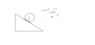# Can the Normal Force Produce a Torque on an Object on an Incline?

rashida564
Hi everyone I can't understand how normal force produce torque isn't normal force acts normally on the plane so the perpendicular distance in case if it was like block will be zero, so normal force doesn't produce torque. "case of box"

Mentor
I don't have any idea what you are describing here. Can you please provide a diagram?

dsaun777
Summary:: Normal force producing torque on inclines.

Hi everyone I can't understand how normal force produce torque isn't normal force acts normally on the plane so the perpendicular distance in case if it was like block will be zero, so normal force doesn't produce torque. "case of box"
Are you referring to internal stress?

rashida564
Is all normal force haze zero torque

#### Attachments

•Untitled.png
3.8 KB · Views: 145
dsaun777
Is all normal force haze zero torque
Depends on the friction I think

Mentor
Is all normal force haze zero torque
Here's a geometry question for you: If you have a line tangent to a circle, the normal to the line at the point of tangency passes through the center of the circle. True of False.

rashida564
Yes

rashida564
But why square shapes get torque from normal forces

Mentor
But why square shapes get torque from normal forces
Please provide a diagram for square shapes.

Mentor
Square
Who says that the normal force results in a torque about the center of the square?

rashida564
How square in friction surface have net torque of zero

dsaun777
How square in friction surface have net torque of zero
Internal "torques" exist in the form of stress.

rashida564
But internal torques comes in pair so they cancel each other

Mentor
As @dsaun777 indicates, if friction is present, the normal force is not distributed uniformly over the base of the square, and the normal stress is greater near the downward edge than near the upper edge on the base.